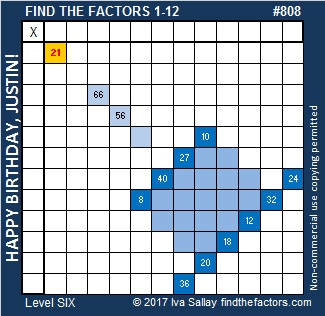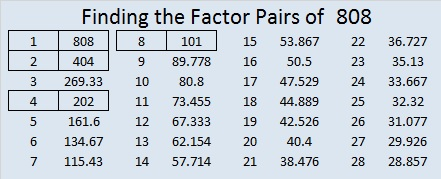# 808 Happy Birthday, Justin!

Happy birthday to my good friend, Justin! He seems to always remember the birthdays of everyone he knows, so this is how I am remembering his special day today. Justin is highly intelligent, thoughtful, and very friendly. I am confident he can solve this Level 6 puzzle that looks a little like a birthday cake.Print the puzzles or type the solution on this excel file: 10-factors 807-814

This is my 808th post so I thought I would also make a factor cake for the number 808. It’s prime factor, 101, is at the top of the cake. Justin, I hope you live to be 101!808 is a palindrome. That means it looks the same forwards and backwards. It is also a strobogrammatic number. That means it looks the same right side up or upside down.

ALL of the factors of 808 are also palindromes, and four of them are strobogrammatic numbers, too. Can you figure out which ones are both?

• 808 is a composite number.
• Prime factorization: 808 = 2 x 2 x 2 x 101, which can be written 808 = (2^3) x 101
• The exponents in the prime factorization are 3 and 1. Adding one to each and multiplying we get (3 + 1)(1 + 1) = 4 x 2 = 8. Therefore 808 has exactly 8 factors.
• Factors of 808: 1, 2, 4, 8, 101, 202, 404, 808
• Factor pairs: 808 = 1 x 808, 2 x 404, 4 x 202, or 8 x 101
• Taking the factor pair with the largest square number factor, we get √808 = (√4)(√202) = 2√202 ≈ 28.425340807Here are the factors that make puzzle #808 act like a multiplication table. It is followed by a table of logical steps to arrive at that solution.